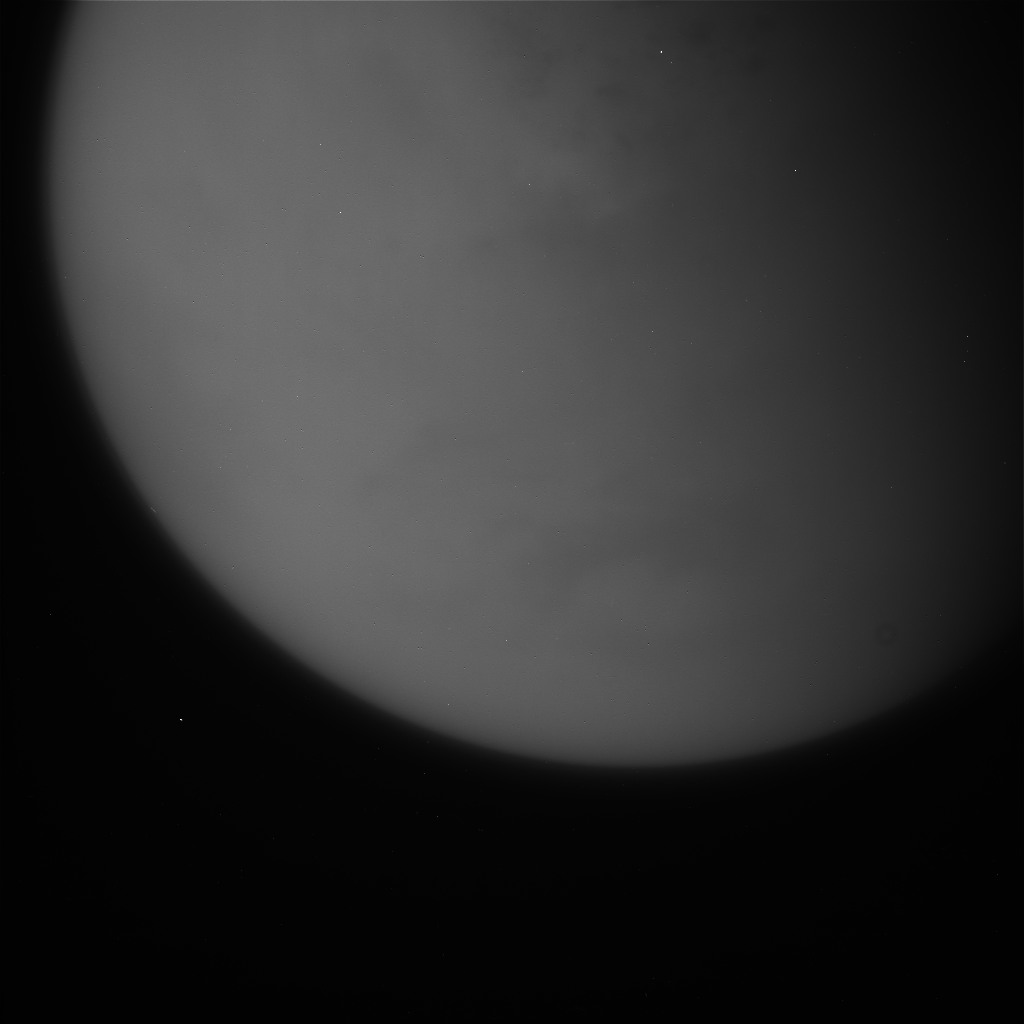## Getting the image data

So, given the information we have from the last step we can extract pixel information. The process is essentially:
• Get the file data
• Skip the label, using the LBLSIZE
• Skip the binary header, using NBB and RECSIZE
• Extract image records - count given by NL * NB (or N2 * N3)
Where each extracted image record is:
• Extract RECSIZE data
• Skip NBB Bytes
• Append what's left to the image data output
This gives us an array of raw pixel data values in the image data output.

So for the case where we have the data in
QByteArray ba;

And we can access the tags we extracted using the method:
raw->GetLabel("foo")

And we have the output image data:
QByteArray im;

Then we can just do:
int datalineskip = raw->GetLabel("NBB").toInt();
int datalinesz = raw->GetLabel("RECSIZE").toInt();
ba = ba.remove(0, raw->GetLabel("LBLSIZE").toInt());

for (int i=0 ; i< raw->GetLabel("NLB").toInt() ; i++)
{
QByteArray imd;
imd = ba.left(datalinesz);
imd.remove(0, datalineskip);
ba.remove(0, datalinesz);
}

int imsz = raw->GetLabel("N2").toInt();
imsz = imsz * raw->GetLabel("N3").toInt();
for (int i=0 ; i< imsz; i++)
{
QByteArray imd;
imd = ba.left(datalinesz);
imd.remove(0, datalineskip);
im.append(imd);
ba.remove(0, datalinesz);
}
And we're done. There's a few assumptions about the way data is organised here, and we're just skipping over the binary header data so we could do that in a simpler manner, but this processing seems to hold up for the records I've used it on.

## Turning it into Pixel Data

Now we have the image data we can turn these into pixel values. In this case, since we're dealing with larger sample sizes than a byte, we'll store them as alist of integers:
QList<int> _data;
This is overkill, since the samples are 16bpp, and practically are actually 12bit values from the DAC, but we're still only dealing with 1024*1024 images at most so this isn't enough for me to worry about. We could use an int16_t or similar if we had to though.

So  our line and sample counts are given by:
int lineCount = _labels["NL"].toInt();
int sampleCount = _labels["NS"].toInt();

And we can get the actual pixel data depth from
if (_labels["FORMAT"] == "'BYTE'")
{
_samplesz = 1;
}
else if (_labels["FORMAT"] == "'HALF'")
{
_samplesz = 2;
}

... else error...

Then we can just form up the data into the output list either directly, or as byte pairs concatenated to 16bpp values:
int cursor =0;
if (_samplesz == 1)
{
for (int y=0; y < lineCount; y++)
for (int x=0; x < sampleCount; x++)
{
if (cursor >= im.size())
return;
_data.append(im.at(cursor++));
}
}
else if (_samplesz == 2)
{
for (int y=0; y < lineCount; y++)
for (int x=0; x < sampleCount; x++)
{
int v;
int vh,vl;

vh = (unsigned char) im.at(cursor);
vl = (unsigned char) im.at(cursor+1);
v = (vh << 8) | vl;
_data.append(v);
cursor +=_samplesz;
if (cursor >= im.size())
{
return;
}
}

}

## Then making a bitmap

Since the basic bitmap types only hold 8bpp we shift down the 16bpp values by 4 to reduce the range when we generate our preview image. This is a bit rubbish, and we really ought to do a proper min/max calculation and level correction  but it'll do for this example. Otherwise this is basically the same as the QImage process we followed previously.
_image = QImage(_width, _height, QImage::Format_RGB32);

cursor =0;
for (int y=0; y < _height; y++)
{
for (int x=0; x < _width; x++)
{
QRgb value;
int v;
v = _data.at(cursor++);
if (_samplesz == 2)
v = v >>4;

value = qRgb(v,v,v);
_image.setPixel(x,y, value);
}
}

sname = _labels["MISSION_NAME"];
sname += " " + _labels["INSTRUMENT_NAME"];
sname += " " + _labels["IMAGE_TIME"];
sname += " " + _labels["TARGET_NAME"];
sname += " " + _labels["FILTER_NAME"];
sname += ".png";

_image.save(sname);

## And the output is

For this case we have the outputFrom Nasa's N1767153499_1.IMG. See Nasa for copyright/usage information.

This is basically the same image you can get from this NASA link, of Titan. However we don't have the image compression artefacts. We're also dimmer thanks to our hacky "shift down by 4", where we could be much smarter about the dynamic range - particularly since the higher values appear to be camera pixel glitches.
Putting the image into something like the gimp and playing with the threshold tools show we could be much better at using the entire dynamic range of the image.

And so onto the next thing: Since the 16 bit grayscale isn't handled well by Qt, and we want to do some more image processing on the raw data, then next time we'll look at replacing our Qt image handler with some OpenCV processing...